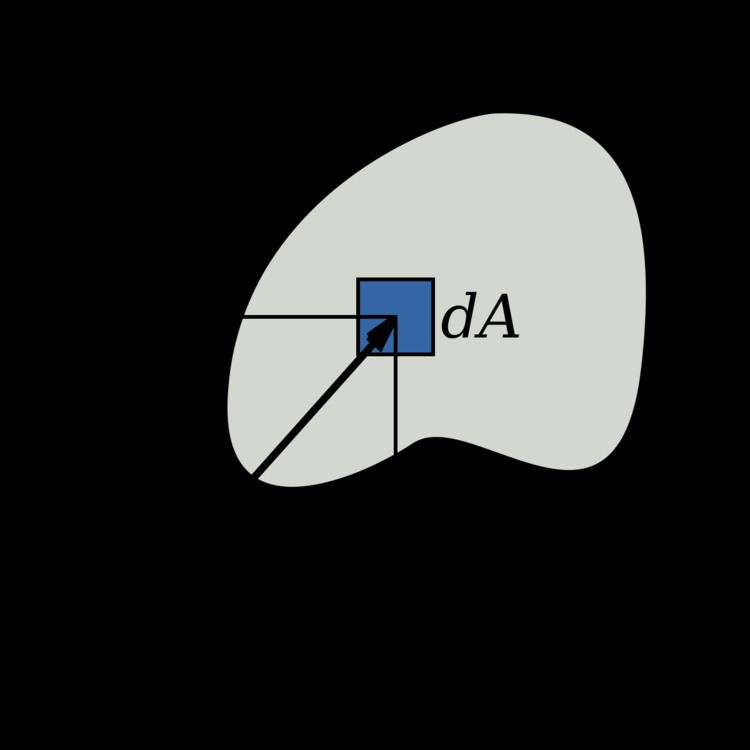# Polar moment of inertia

Updated on
Covid-19Polar moment of inertia is a quantity used to predict an object's ability to resist torsion, in objects (or segments of objects) with an invariant circular cross section and no significant warping or out-of-plane deformation. It is used to calculate the angular displacement of an object subjected to a torque. It is analogous to the area moment of inertia, which characterizes an object's ability to resist bending and is required to calculate displacement.

## Contents

The larger the polar moment of area, the less the beam will twist, when subjected to a given torque.

Polar moment of area should not be confused with moment of inertia, which characterizes an object's angular acceleration due to a torque. See moment (physics).

Note: It has become common to use "Moment of Inertia" (MOI) to refer to either or both of the planar second moment of area, I = A x 2 d A , where x is the distance to some reference plane, or the polar second moment of area, I = A r 2 d A , where r is the distance to some reference axis. In each case the integral is over all the infinitesimal elements of area, dA, in some two-dimensional cross-section. "Moment of Inertia" is, strictly, the second moment of mass with respect to distance from an axis: I = m r 2 d m , where r is the distance to some potential rotation axis, and the integral is over all the infinitesimal elements of mass, dm, in a three-dimensional space occupied by an object. The MOI, in this sense, is the analog of mass for rotational problems.

## Limitations

The polar moment of area cannot be used to analyze shafts with non-circular cross-sections. In such cases, the torsion constant can be substituted instead.

In objects with significant cross-sectional variation(along the axis of the applied torque), which cannot be analyzed in segments, a more complex approach may have to be used. See 3-D elasticity.

However the polar moment of area can be used to calculate the moment of inertia of an object with arbitrary cross-section.

## Definition

I z = A ρ 2 d A
• Iz = the polar moment of area about the axis z
• dA = an elemental area
• ρ = the radial distance to the element dA from the axis z

• For a circular section with radius r:

I z = 0 2 π 0 r ρ 2 ρ d ρ d ϕ = π r 4 2

## Unit

The SI unit for polar moment of area, like the area moment of inertia, is metre to the fourth power (m4).

## Conversion from Area Moment of Inertia

By the perpendicular axis theorem, the following equation relates Iz to the area moments of inertia about the other two mutually perpendicular axes:

I z = I x + I y

## Application

NOTE: The following is only true for a circular or hollow circular section.

The polar moment of area appears in the formulae that describe torsional stress and angular displacement.

Torsional stress:

τ = T r J z

where T is the torque, r is the distance from the center and J z is the polar moment of area.

In a circular shaft, the shear stress is maximal at the surface of the shaft.

## Sample calculation

Calculation of the steam turbine shaft radius for a turboset:

Assumptions:

• Power carried by the shaft is 1000 MW; this is typical for a large nuclear power plant.
• Yield stress of the steel used to make the shaft (τyield) is: 250 x 106 N/m².
• Electricity has a frequency of 50 Hz; this is the typical frequency in Europe. In North America the frequency is 60 Hz. This is assuming that there is a 1:1 correlation between rotational velocity of turbine and the frequency of mains power.
• The angular frequency can be calculated with the following formula:

ω = 2 π f

The torque carried by the shaft is related to the power by the following equation:

P = T ω

The angular frequency is therefore 314.16 rad/s and the torque 3.1831 x 106 N·m.

The maximal torque is:

T max = τ max J z r

After substitution of the polar moment of inertia the following expression is obtained:

r = 2 T max π τ max 3

The radius is 0.200 m. If one adds a factor of safety of 5 and re-calculates the radius with the maximal stress equal to the yield stress/5 the result is a radius of 0.343 m, or a diameter of 69 cm, the approximate size of a turboset shaft in a nuclear power plant.

## Polar moment of inertia

Hollow cylinder

I z = π ( D 4 d 4 ) 32

Moment of inertia

J z = I z ρ l = π ρ l ( D 4 d 4 ) 32

Solid Cylinder

I z = π D 4 32

Moment of inertia

J z = I z ρ l = π ρ l D 4 32

where:

• d is the inner diameter in m
• D is the outside diameter in m
• J z is the moment of inertia in kg m 2
• l is the length of cylinder in m
• ρ is the specific mass in kg/m 3
• ## References

Similar Topics
The Rivers End (film)
Rob Briley
Peta Sergeant
Topics